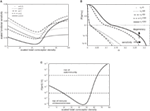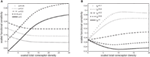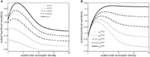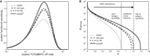Impact Factor 5.085 | CiteScore 5.4
More on impact ›

# Frontiers in Immunology

## General Commentary ARTICLE

Front. Immunol., 16 September 2014 | https://doi.org/10.3389/fimmu.2014.00443

# Corrigendum: Co-receptor CD8-mediated modulation of T-cell receptor functional sensitivity and epitope recognition degeneracy

• 1University of Warwick, Coventry, UK
• 2Institute of Infection and Immunity, Cardiff University School of Medicine, Cardiff, UK
• 3Faculty of Medical and Veterinary Sciences, University of Bristol, Bristol, UK

A corrigendum on

Co-receptor CD8-mediated modulation of T-cell receptor functional sensitivity and epitope recognition degeneracy
by Szomolay B, Williams T, Wooldridge L, van den Berg HA. Front Immunol (2013) 4:329. doi: 10.3389/fimmu.2013.00329

It has been kindly pointed out to us by Dr. Omer Dushek of Oxford University that the thermodynamic constraints (arising from the principle of detailed balance) impose the following condition on the parameters:

$ν=γkinδ$

which means that the parameter ν is fixed once γkin and δ have each been assigned a value. The objective of the paper was to exhibit the range of qualitative behaviors that is possible when pMHCI/CD8 kinetics interacts with TCR/pMHCI kinetics and to show how varying levels of the co-receptor at the T-cell surface may be able to modulate the functional sensitivity of the T-cell to various ligands in a differential fashion. These qualitative phenomena remain very much the same when we choose parameter values that respect the constraint ν = γkinδ, as shown in the corrected figures that follow below (Figures 24). It is these qualitative patterns that are currently guiding experimental research to elucidate CD8-mediated ligand focusing in the T-cell system. The main thrust of the paper is therefore unaltered.

FIGURE 2Figure 2. (A) Scaled functional sensitivity w as a function of scaled total CD8 density xT for various scaled TCR/pMHCI off-rates α. (B) Degeneracy curves ℙ(w > ω) for various scaled total CD8 density xT. (C) The probability ℙ(w > ω) as a function of CD8 density xT, at a set value of functional sensitivity ω = 0.14. The operating range of the probability ℙ is shown as a function of xT with dashed lines at ℙ(w > 0.14) = 10−8 and ℙ(w > 0.14) = 10−5. Parameters are as follows: $δ=300,n=100,γkin=0.1,γoff=0.5,γR=1,κ=5.5,mT=10,rT=10$. The log-normal distribution has mean 2 and SD 0.2. Changed: ω = 0.14, γkin, γR,κ and α’s in (A).

FIGURE 3Figure 3. Scaled functional sensitivity w as a function of scaled total CD8 density xT. (A) Curves for various values of the scaled TCR/pMHCI off-rate α. (B) Curves for various values of the factor γR by which CD8 modulates the TCR triggering threshold. Parameters, unless stated otherwise, are as follows: δ = 2. 5, n = 100, γkin = 0. 5, γoff = 0. 5, γR = 0. 7, κ = 1, mT = 10, rT = 10, α = 1. Changed: γR.

FIGURE 4Figure 4. Scaled functional sensitivity w as a function of scaled total CD8 density xT. (A) Curves for various values of the scaled TCR/pMHCI off-rate α. (B) Curves for various values of the dimensionless factor γoff expressing the modulatory effect of CD8 on the TCR/pMHCI off-rate. Parameters, unless stated otherwise, are as follows: δ = 500, n = 100, γkin = 0. 2, γoff = 0. 2, γR = 0. 01, κ = 1, mT = 10, rT = 10, α = 1. Changed: δ, γkin, γR,α,κ, and α’s in (A) and γoff’s in (B).

FIGURE 5Figure 5. (A) Scaled functional sensitivity w as a function of scaled TCR/pMHCI off-rate α. (B) Degeneracy curves ℙ(w > ω) for HLA A*0201 mutants with altered binding affinity for CD8: A245V (dotted line), wild-type (semi-dashed line), Q115E (dashed line), and A2/α3kb (solid line). The three regions represent the overall pattern of CD8+ T-cell antigen specificity and the arrow indicates the strength of pMHCI/CD8 interaction. Wild-type parameters are as follows: δ = 0.2, n = 100, γkin = 0. 5, γoff = 0. 5, γR = 0. 2, κ = 1, mT = 10, rT = 10, xT = 10; the parameter δ is adjusted in proportion to the ratio between the mutant pMHCI/CD8 affinity constant (i.e., dissociation constant) and the wild-type affinity, whereas the parameters κ and xT are adjusted in inverse proportion to the mutant/wild-type pMHCI/CD8 affinity (these adjustments follow from the scaling). The log-normal distribution has mean 5 and SD 0.5. Figure 5C has been deleted.

The kinetic scheme (Figure 1) and the equations are all unaltered, except for a typographical error in the subscript of a quantity appearing in one of the equations. Specifically, equation (23) in the published text should read:

$W=MRλ−1P00+MXRλ−4P0∗.$

We apologize profusely for any inconvenience our oversight may have caused.

## Conflict of Interest Statement

The authors declare that the research was conducted in the absence of any commercial or financial relationships that could be construed as a potential conflict of interest.

Keywords: corrigendum, T cell, T cell receptor, T cell repertoire, T cell response

Citation: Szomolay B, Williams T, Wooldridge L and van den Berg HA (2014) Corrigendum: Co-receptor CD8-mediated modulation of T-cell receptor functional sensitivity and epitope recognition degeneracy. Front. Immunol. 5:443. doi: 10.3389/fimmu.2014.00443

Received: 06 August 2014; Accepted: 31 August 2014;
Published online: 16 September 2014.

Edited by:

Bruno Laugel, Cardiff University School of Medicine, UK

Reviewed by:

Brian M. Baker, University of Notre Dame, USA
Yoram Louzoun, Bar-Ilan University, Israel

Copyright: © 2014 Szomolay, Williams, Wooldridge and van den Berg. This is an open-access article distributed under the terms of the Creative Commons Attribution License (CC BY). The use, distribution or reproduction in other forums is permitted, provided the original author(s) or licensor are credited and that the original publication in this journal is cited, in accordance with accepted academic practice. No use, distribution or reproduction is permitted which does not comply with these terms.

*Correspondence: hugo@maths.warwick.ac.uk